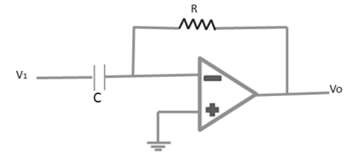# Semiconductor Devices - Differentiator

A differentiator circuit is shown in the following figure.The differentiator provides a useful operation, the resulting relation for the circuit being

Vo(t) = RC(dv1(t)/dt

Following are some important parameters of Operational amplifier −

## Open Loop Voltage Gain (AVOL)

The open loop voltage gain of an operational amplifier is its differential gain under conditions where no negative feedback is used. AVOL ranges from 74 db to 100 db.

AVOL = [Vo/(V1 – V2)]

## Output Offset Voltage (VOO)

The output offset voltage of an operational amplifier is its output voltage when its differential input voltage is zero.

## Common Mode Rejection (CMR)

If both the inputs are at the same potential, causing the differential input zero, and if the output is zero, the operational amplifier is said to have a good common mode rejection.

## Common Mode Gain (AC)

Common mode gain of an operational amplifier is the ratio of the common mode output voltage to the common mode input voltage.

The differential gain of an operational amplifier is the ratio of the output to the differential input.

Ad = [Vo / (V1) - V2]

## Common Mode Rejection Ratio (CMRR)

CMRR of an operational amplifier is defined as the ratio of the closed loop differential gain to the common mode gain.

## Slew Rate (SR)

Slew rate is the rate of output voltage change caused by a step input voltage. An ideal slew rate is infinite, which means that the operational amplifier output should change instantly in response to an input step voltage.

We have already discussed some applications of op-amp such as differentiator, integrator, summing amplifier, etc. Some other common applications of operational amplifiers are −

• Logarithmic amplifier
• Gyrator (Inductance simulator)
• DC & AC voltage follower
• Analog to Digital converter
• Digital to Analog converter
• Power supplies for over voltage protection
• Polarity indicator
• Voltage follower
• Active filters